### Abstract:

In this paper I prove with algebra and propositional logic that problems (represented as information in a computer system or the the superset p) can theoretically be solved over time by Artificial Intelligence. I do this by merging relational algebra / set theory from mathematics (mathematical proof/s), nondeterministic Quantum Physics and computing theory from theoretical physics (conceptually) and finally using propositional logic for the final proof to solve the problem P=NP over polynomial time(t) and propose a new law of Quantum Computing -” T he Quantum Law of pre-processing”

### Notes belowChaos States in information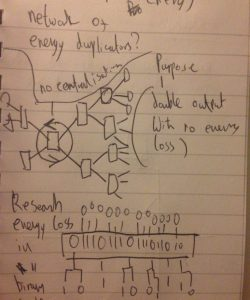Energy Duplicators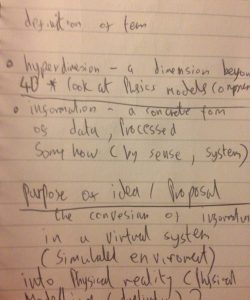Description of Terms.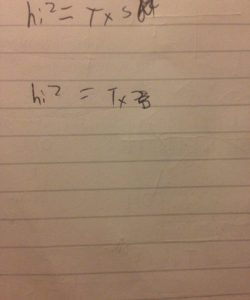Rough ideas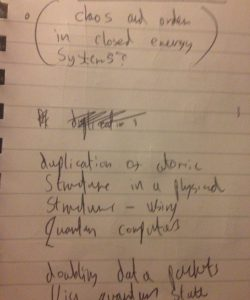Duplicating atomic structures and entropy -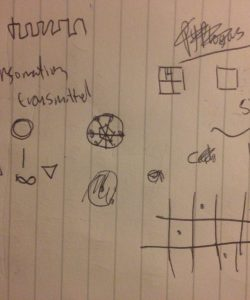Sketching visual ideas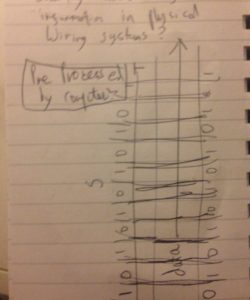Pre-Processor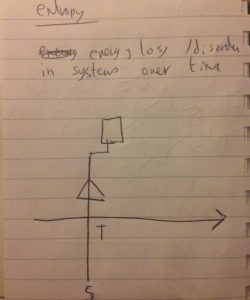Energy loss / disorder in systems over time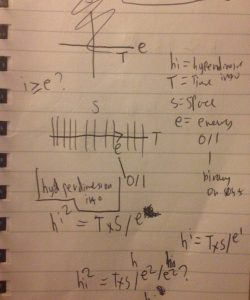Intuitions on hyper-dimension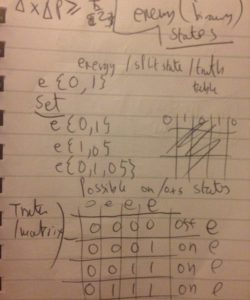On / off binart states split states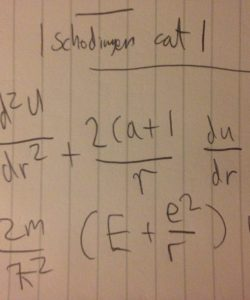Cat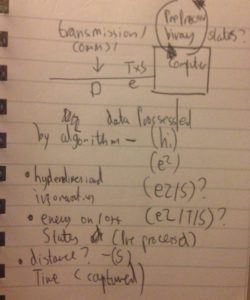Hyper-dimensional Information